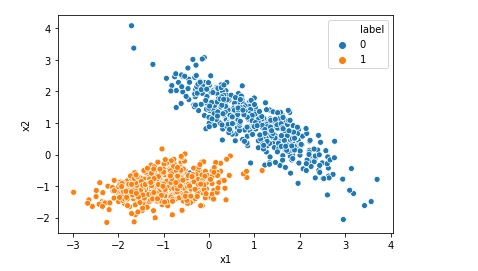# Difference between Logistic Regression and Support Vector Machine SVM

Both Logistic Regression and Support Vector Machine or SVM can be used for classification however there is some fundamental difference between them. Lets understand those differences when we are using Support Vector Machine as a classifier. Logistic Regression gives probabilities as output that can be interpreted as confidence in the classification decision however Support Vector … Continue reading Difference between Logistic Regression and Support Vector Machine SVM